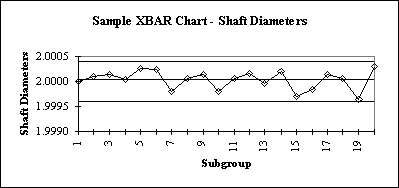Theoretical Control Limits for XBAR Charts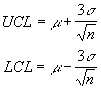Although theoretically possible, since we do not know either the population process mean or standard deviation, these formulas cannot be used directly and both must be estimated from the process itself. First the s chart is constructed. If the s chart validates that the process variation is in statistical control, the XBAR chart is constructed.

Steps in Constructing an s Chart

1. Select k successive subgroups where k is at least 20, in which there are n measurements in each subgroup. Typically n is between 1 and 9. 3, 4, or 5 measurements per subgroup is quite common.
2. Find the sample standard deviation of each subgroup s(i).
3. Find the centerline for the s chart, denoted by
4.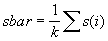5. Find the UCL and LCL with the following formulas: UCL= B(4)SBAR and LCL=B(3)SBAR with B(3) and B(4) can be found in the following table:
6. `Table of B(3) and B(4)`
`n    B(3)    B(4)       n    B(3)    B(4)`
`2     0      3.267      6    .03     1.970`
`3     0      2.568      7    .118    1.882`
`4     0      2.266      8    .185    1.815`
`5     0      2.089      9    .239    1.761`
7. Plot the subgroup data and determine if the process is in statistical control. If not, determine the reason for the assignable cause, eliminate it, and the subgroup(s) and repeat the previous 3 steps. Do NOT eliminate subgroups with points out of range for which assignable causes cannot be found.
8. Once the s chart is in a state of statistical control and the centerline SBAR can be considered a reliable estimate of the range, the process standard deviation can be estimated using:
9.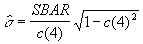c(4) can be found in the following table:

`n  c(4)     n   c(4)`
`2  .7979    6   .9515`
`3  .8862    7   .9594`
`4  .9213    8   .9650`
`5  .9400    9   .9693`

Steps in Constructing the XBAR Chart

1. Find the mean of each subgroup XBAR(1), XBAR(2), XBAR(3)… XBAR(k) and the grand mean of all subgroups using:
2.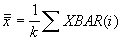3. Find the UCL and LCL using the following equations:
4.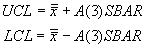`A(3) can be found in the following table:`
`n   A(3)     n   A(3)`
`2   2.659    6   1.287`
`3   1.954    7   1.182`
`4   1.628    8   1.099`
`5   1.427    9   1.032`
5. Plot the LCL, UCL, centerline, and subgroup means
6. Interpret the data using the following guidelines to determine if the process is in control:
7. `a.  one point outside the 3 sigma control limits`
`b.  eight successive points on the same side of the centerline`
`c.  six successive points that increase or decrease`
`d.  two out of three points that are on the same side of the centerline, both at a distance exceeding 2 sigmas from the centerline`
`e.  four out of five points that are on the same side of the centerline, four at a distance exceeding 1 sigma from the centerline`
`f.  using an average run length (ARL) for determining process anomalies`

Example:

The following data consists of 20 sets of three measurements of the diameter of an engine shaft.

```n	meas#1	meas#2	meas#3		StdDev	XBAR
1	2.0000	1.9998	2.0002		0.0002	2.0000
2	1.9998	2.0003	2.0002		0.0003	2.0001
3	1.9998	2.0001	2.0005		0.0004	2.0001
4	1.9997	2.0000	2.0004		0.0004	2.0000
5	2.0003	2.0003	2.0002		0.0001	2.0003
6	2.0004	2.0003	2.0000		0.0002	2.0002
7	1.9998	1.9998	1.9998		0.0000	1.9998
8	2.0000	2.0001	2.0001		0.0001	2.0001
9	2.0005	2.0000	1.9999		0.0003	2.0001
10	1.9995	1.9998	2.0001		0.0003	1.9998
11	2.0002	1.9999	2.0001		0.0002	2.0001
12	2.0002	1.9998	2.0005		0.0004	2.0002
13	2.0000	2.0001	1.9998		0.0002	2.0000
14	2.0000	2.0002	2.0004		0.0002	2.0002
15	1.9994	2.0001	1.9996		0.0004	1.9997
16	1.9999	2.0003	1.9993		0.0005	1.9998
17	2.0002	1.9998	2.0004		0.0003	2.0001
18	2.0000	2.0001	2.0001		0.0001	2.0001
19	1.9997	1.9994	1.9998		0.0002	1.9996
20	2.0003	2.0007	1.9999		0.0004	2.0003

SBAR CHART LIMITS:
SBAR  =	0.0002

UCL   =	 B(4)*SBAR   =	2.568*.0002 =	0.0005136
LCL   =  B(3)*SBAR   =    0 * .0002 =	0.00

XBAR CHART LIMITS:
XDBLBAR  =		2.0000

UCL  =	XDBLBAR + A(3)*SBAR   =	2.000+1.954*.0002  = 2.0003908
LCL   =	XDBLBAR - A(3)*SBAR   =	2.000-1.954*.0002  = 1.9996092
```

s – Chart: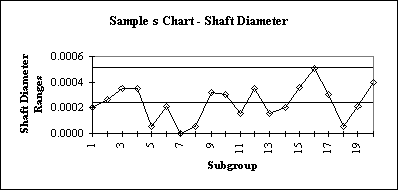XBAR – Chart: